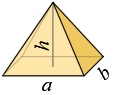### Volume and Surface Area of Rectangular PyramidDimensions of base

a = , b =

Height

h =

Volume

V = 13·a·b·h

Area of side faces

So = a· h2 + b24 + b· h2 + a24

Area of base

A = a·b

Total surface area

S = So + A